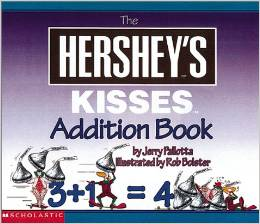9 teachers like this lesson
Print Lesson

Objective

SWBAT solve adding to and putting together situation using the strategy of drawing a picture.

Big Idea

Letâs put it all together! In this lesson students will use addition strategies to solve word problems.

Activating Strategy

10 minutesThere is also a You Tube version, if the book isn't available.

I then write the following problem on the board, or chart paper.  (Also available as a PPT Addition Strategies)

Sam picked 5 apples.  Sue picked 4 apples.   How many apples did they pick?

I have student volunteers come to the board to demonstrate how they solve the addition problem.

In this video, the student is using the strategy of drawing a picture to solve the problem.

Teaching Strategies

15 minutes

I then write the following problem on the board or chart paper (also available as a PPT Addition Strategies)

Megan put 8 fish in the tank.  Tess put in 2 more fish.  Then Bob put in 3 more fish.  How many fish are in the tank now?

I guide the discussion by asking the following questions:

• What do you need to find? (How many fish are in the tank now.)
• What numbers to you need to add? (8, 2, and 3.)
• Which two numbers will you add first? Why?  (I will add 8 and 2 first to make ten. I can use a ten frame to add any number plus ten.)
• How can using a ten frame help you solve the problem? (I can draw use a ten frame to show 8 + 2, then add 3 more to show 10 + 3.)

I then work through the problem together with students to model how to add three numbers.

To get the students ready for the independent practice portion, I make sure that students have counters to model the following problem:

Mark has 9 green toy cars.  He has 1 yellow toy car.  He has 5 blue cars.  How many toy cars does he have?

I then guide the discussion by asking the following questions:

• What do you need to find? (How many toy cars Mark has.)
• What information do you need to use? (He has 9 green toy cars, 1 yellow car and 5 blue cars.)
• Which two numbers would you add first? Why?  (9 and 1.  I can use a ten frame to add any number plus ten.)

I encourage students to use a strategy that they are comfortable with to solve the problem.

Independent Practice

30 minutes

For the independent practice portion of this lesson, I like to have students complete Addition strategies worksheet.  This worksheet allows the students multiple opportunities to practice their addition strategies of drawing a picture and counting on to solve word problems.  They are also given the opportunity to analyze the word problem to determine what information is needed to solve the problem.

For struggling students, instead of them drawing a picture, I encourage them to use manipulatives.

Closing/Summarizing

5 minutes

To close out the lesson, I like to have the students use their math journals to draw a picture to show how they would solve this problem:

I have 8 red blocks and 4 blue blocks.  How many blocks do I have?